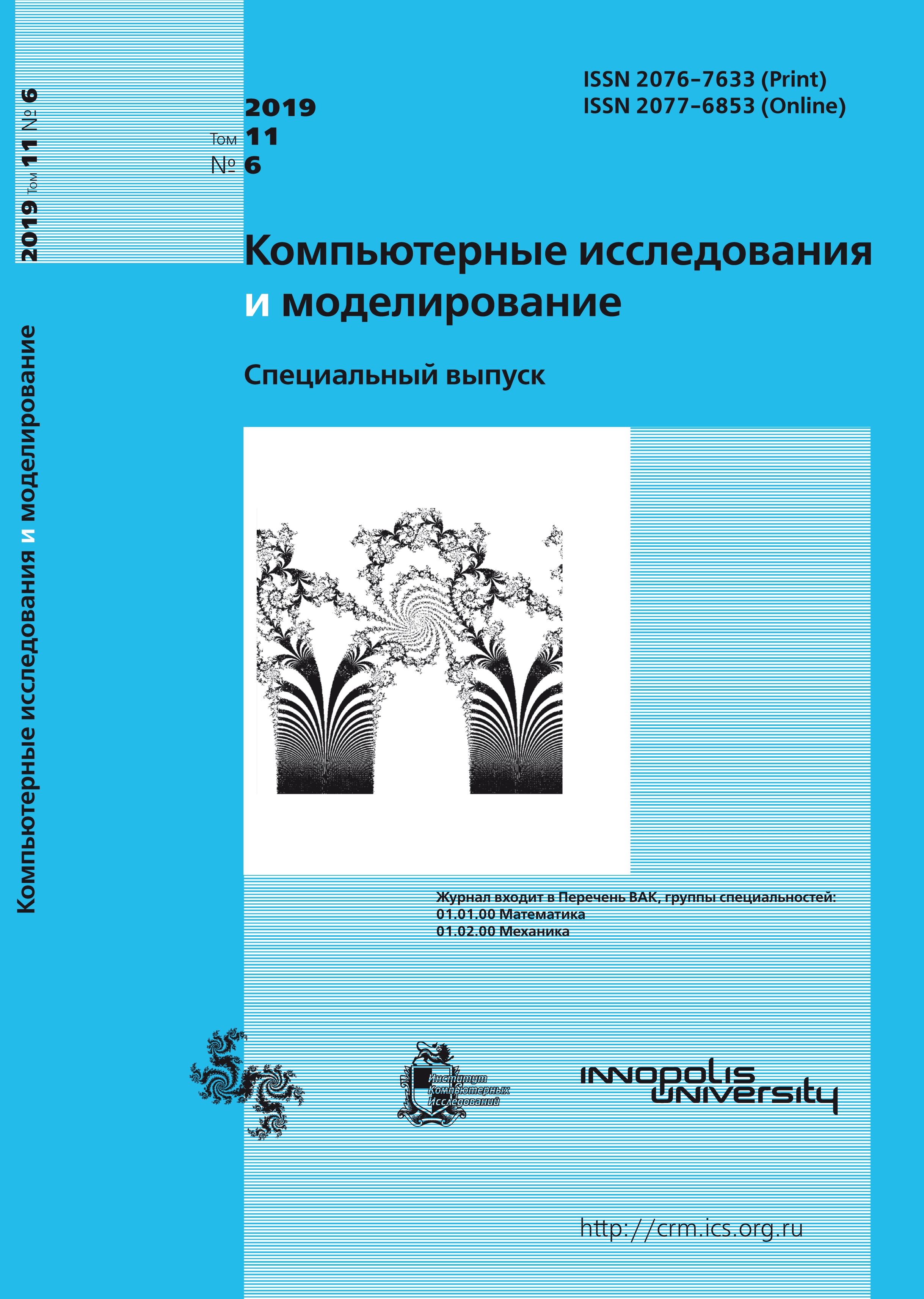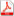Issue 6, 2019 Vol. 11

# All issues

Linear and nonlinear optimization models of multiple covering of a bounded plane domain with circlespdf (111K)

Problems of multiple covering ($k$-covering) of a bounded set $G$ with equal circles of a given radius are well known. They are thoroughly studied under the assumption that $G$ is a finite set. There are several papers concerned with studying this problem in the case where $G$ is a connected set. In this paper, we study the problem of minimizing the number of circles that form a $k$-covering, $k \geqslant 1$, provided that $G$ is a bounded convex plane domain.

For the above-mentioned problem, we state a 0-1 linear model, a general integer linear model, and a nonlinear model, imposing a constraint on the minimum distance between the centers of covering circles. The latter constraint is due to the fact that in practice one can place at most one device at each point. We establish necessary and sufficient solvability conditions for the linear models and describe one (easily realizable) variant of these conditions in the case where the covered set $G$ is a rectangle.

We propose some methods for finding an approximate number of circles of a given radius that provide the desired $k$-covering of the set $G$, both with and without constraints on distances between the circles’ centers. We treat the calculated values as approximate upper bounds for the number of circles. We also propose a technique that allows one to get approximate lower bounds for the number of circles that is necessary for providing a $k$-covering of the set $G$. In the general linear model, as distinct from the 0-1 linear model, we require no additional constraint. The difference between the upper and lower bounds for the number of circles characterizes the quality (acceptability) of the constructed $k$-covering.

We state a nonlinear mathematical model for the $k$-covering problem with the above-mentioned constraints imposed on distances between the centers of covering circles. For this model, we propose an algorithm which (in certain cases) allows one to find more exact solutions to covering problems than those calculated from linear models.

For implementing the proposed approach, we have developed computer programs and performed numerical experiments. Results of numerical experiments demonstrate the effectiveness of the method.

Keywords: linear models of the multiple covering problem, $k$-covering of a bounded set, nonlinear models of the $k$-covering problem with circles of a given radius, solvability conditions for linear models of the $k$-covering problem
Citation in English: Khorkov A.V., Khorkov A.V. Linear and nonlinear optimization models of multiple covering of a bounded plane domain with circles // Computer Research and Modeling, 2019, vol. 11, no. 6, pp. 1101-1110
Citation in English: Khorkov A.V., Khorkov A.V. Linear and nonlinear optimization models of multiple covering of a bounded plane domain with circles // Computer Research and Modeling, 2019, vol. 11, no. 6, pp. 1101-1110
DOI: 10.20537/2076-7633-2019-11-6-1101-1110

Full-text version of the journal is also available on the web site of the scientific electronic library eLIBRARY.RU

The journal is included in the Russian Science Citation Index

The journal is included in the List of Russian peer-reviewed journals publishing the main research results of PhD and doctoral dissertations.

International Interdisciplinary Conference "Mathematics. Computing. Education"

The journal is included in the RSCI

Indexed in Scopus